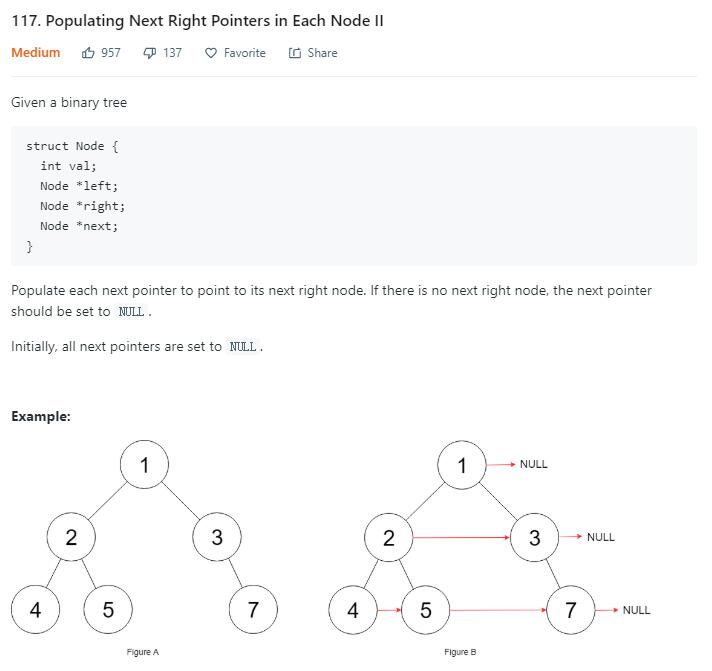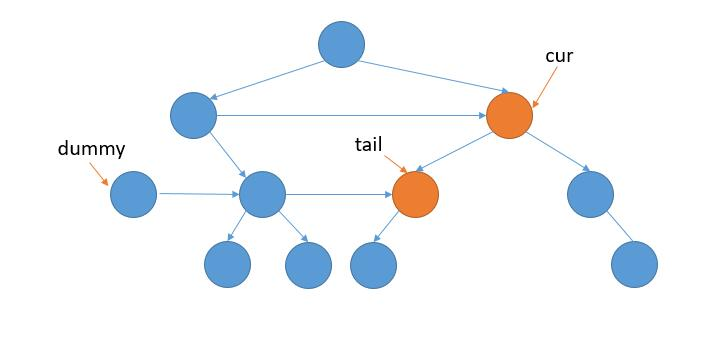# 题目描述（中等难度）# 解法一 BFS

public Node connect(Node root) {
if (root == null) {
return root;
}
queue.offer(root);
while (!queue.isEmpty()) {
int size = queue.size();
Node pre = null;
for (int i = 0; i < size; i++) {
Node cur = queue.poll();
if (i > 0) {
pre.next = cur;
}
pre = cur;
if (cur.left != null) {
queue.offer(cur.left);
}
if (cur.right != null) {
queue.offer(cur.right);
}

}
}
return root;
}


# 解法二

Node connect(Node root) {
if (root == null)
return root;
Node pre = root;
Node cur = null;
while (pre.left != null) {
cur = pre;
while (cur != null) {
cur.left.next = cur.right;
if (cur.next != null) {
cur.right.next = cur.next.left;
}
cur = cur.next;
}
pre = pre.left;
}

return root;
}


cur.left.next = cur.right;
cur.right.next = cur.next.left;


while (cur.left == null && cur.right == null) {
cur = cur.next;
}


Node connect(Node root) {
if (root == null)
return root;
Node pre = root;
Node cur = null;
while (true) {
cur = pre;
while (cur != null) {
//找到至少有一个孩子的节点
if (cur.left == null && cur.right == null) {
cur = cur.next;
continue;
}
//找到当前节点的下一个至少有一个孩子的节点
Node next = cur.next;
while (next != null && next.left == null && next.right == null) {
next = next.next;
if (next == null) {
break;
}
}
//当前节点的左右孩子都不为空，就将 left.next 指向 right
if (cur.left != null && cur.right != null) {
cur.left.next = cur.right;
}
//要接上 next 的节点的孩子，所以用 temp 处理当前节点 right 为 null 的情况
Node temp = cur.right == null ? cur.left : cur.right;

if (next != null) {
//next 左孩子不为 null，就接上左孩子。
if (next.left != null) {
temp.next = next.left;
//next 左孩子为 null，就接上右孩子。
} else {
temp.next = next.right;
}
}

cur = cur.next;
}
//找到拥有孩子的节点
while (pre.left == null && pre.right == null) {
pre = pre.next;
//都没有孩子说明已经是最后一层了
if (pre == null) {
return root;
}
}
//进入下一层
pre = pre.left != null ? pre.left : pre.right;
}
}


# 解法三

if (cur.left != null) {
queue.offer(cur.left);
}
if (cur.right != null) {
queue.offer(cur.right);
}cur 指针利用 next 不停的遍历当前层。

curnull 的时候，再利用 dummy 指针得到新的一层的开始节点。

dummy 指针在链表中经常用到，他只是为了处理头结点的情况，它并不属于当前链表。

Node connect(Node root) {
Node cur = root;
while (cur != null) {
Node dummy = new Node();
Node tail = dummy;
//遍历 cur 的当前层
while (cur != null) {
if (cur.left != null) {
tail.next = cur.left;
tail = tail.next;
}
if (cur.right != null) {
tail.next = cur.right;
tail = tail.next;
}
cur = cur.next;
}
//更新 cur 到下一层
cur = dummy.next;
}
return root;
}